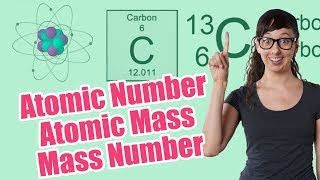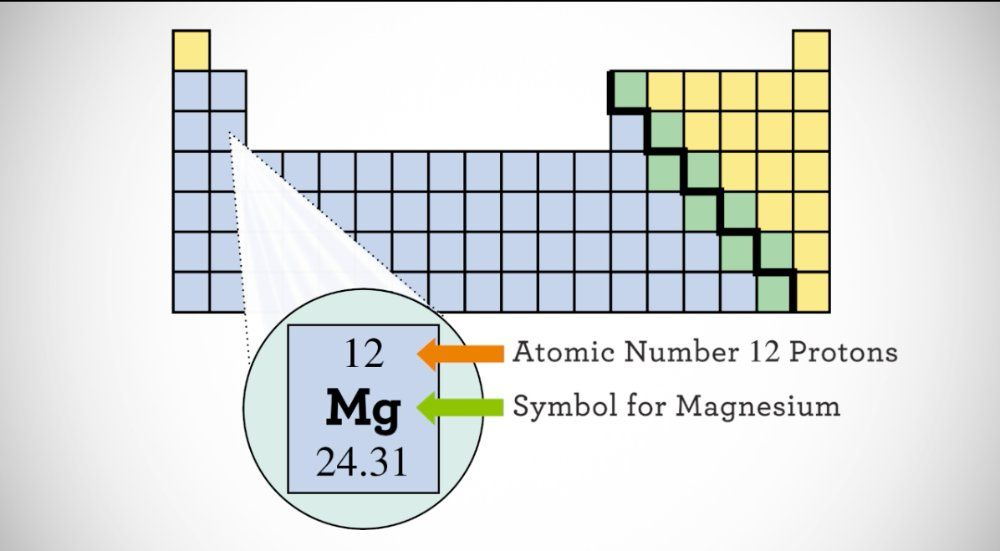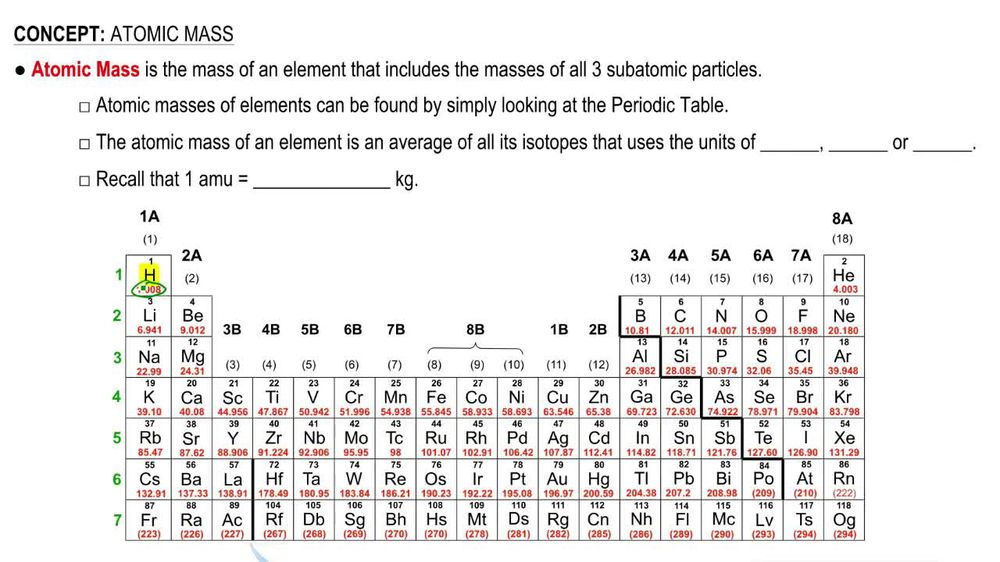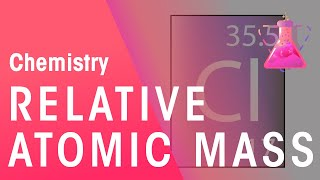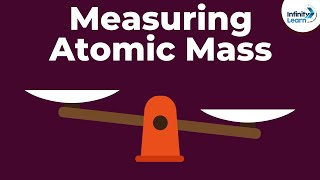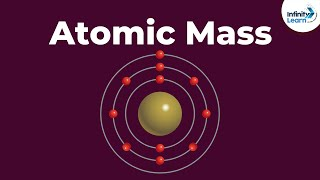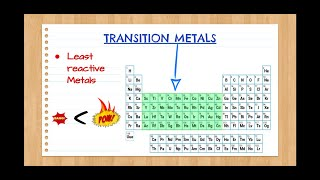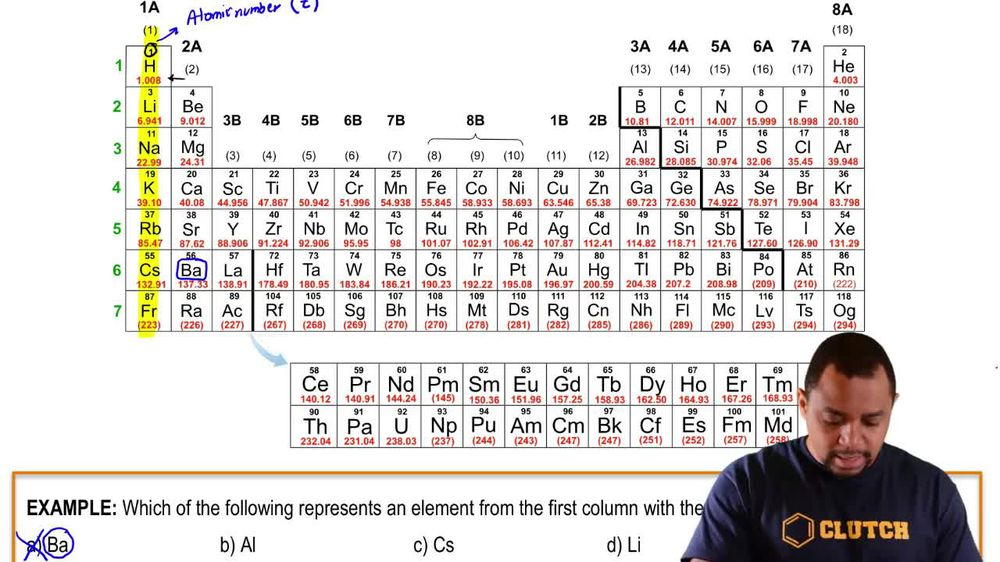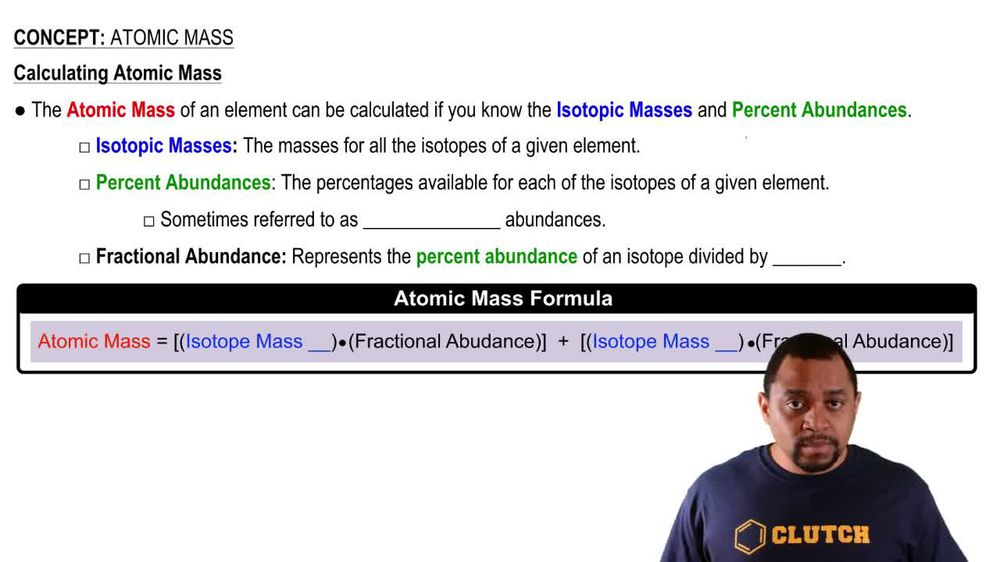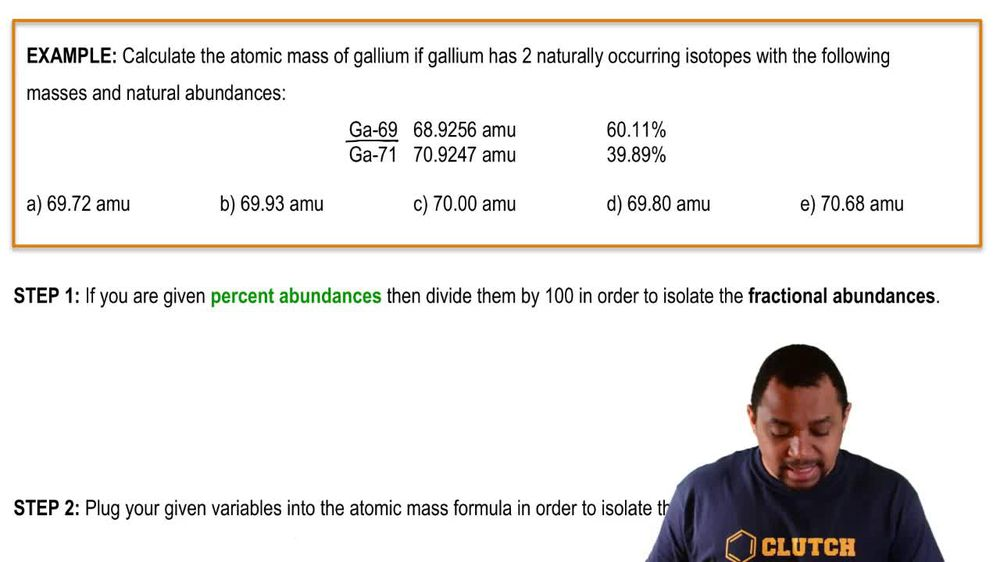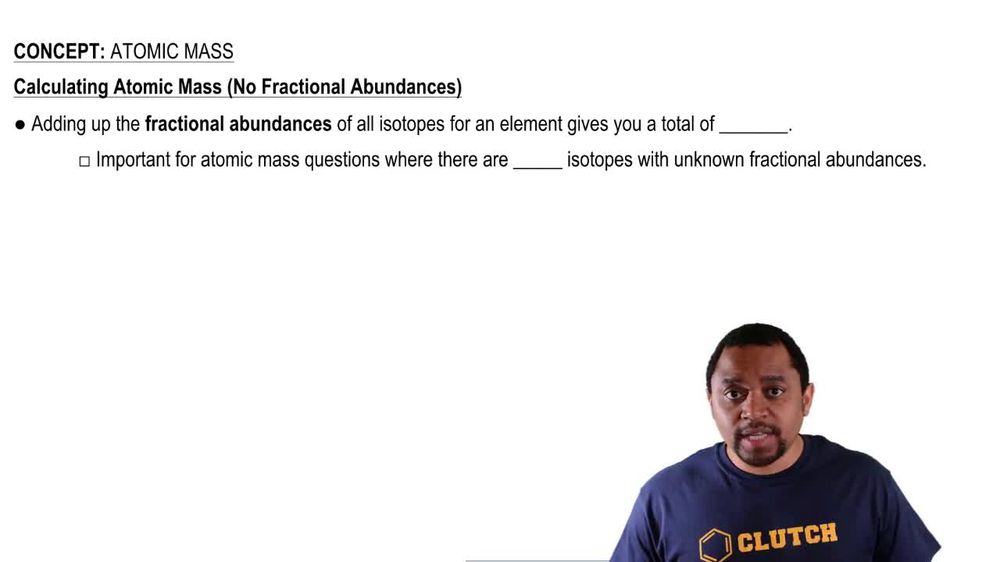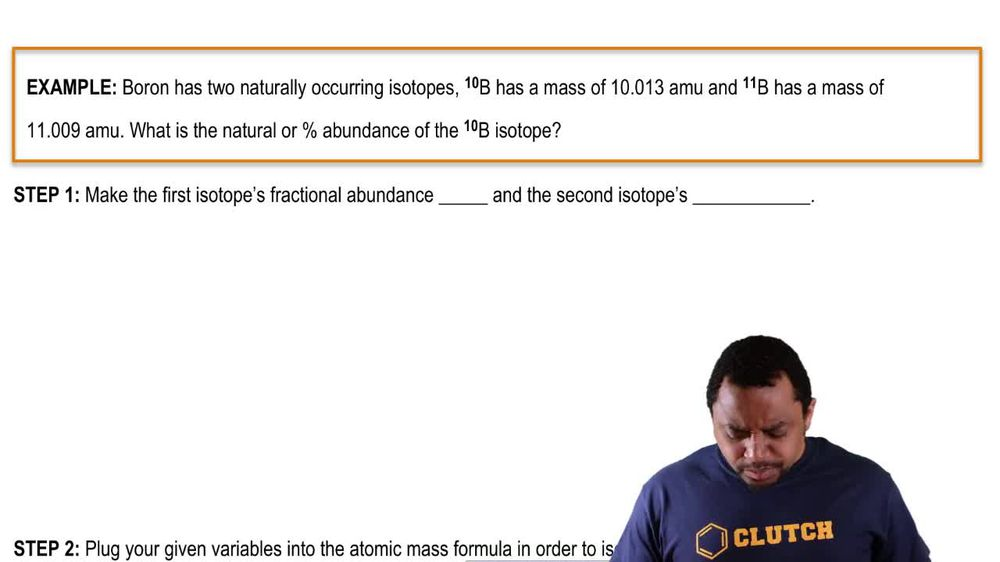Start typing, then use the up and down arrows to select an option from the list.
1. 2. Atoms & Elements2. Atomic Mass
Problem

# A sample of naturally occurring silicon consists of 28^Si (27.9769), 29^Si (28.9765), and 30^Si (29.9738). If the atomic weight of silicon is 28.0855 and the natural abundance of 29^Si is 4.68%, what are the natural abundances of 28^Si and 30^Si?

Relevant Solution9m
Play a video:
Hi everyone for this problem, it reads the atomic weight of magnesium is 24. atomic mass units. The natural isotopes of magnesium are magnesium 24. Magnesium 25 magnesium 26. If the natural abundance of magnesium 26 is 11% calculate the natural abundance Of the remaining isotopes. Okay, so we want to calculate the natural abundance of the remaining isotopes. And then the problem we know what the natural abundance is of Magnesium 26. It's 11%. So that means we need to now figure out the natural abundance for Magnesium 24 and 25. So let's just recall that atomic weight is the weighted average of the mass of natural isotope by their abundance. Okay, so here let's use some substitution here. So we'll define X as the abundance Of Magnesium 24. And we'll define why as the abundance Of Magnesium 25. Okay, so what we know is that Is going to equal these three isotopes. Magnesium 24, and 26. So we'll say X. Which is magnesium 24 plus Y, which is magnesium 25 plus 11%. Which is magnesium 26. Okay, so Let's isolate this equation so that we're solving for one variable. So let's choose why as our variable. So when we write this we're going to get why is equal to 100 minus X -11%. Okay, so that means why if we take 100% -11%, that is 89%. So we have 89% -1 is going to be Y. All right. And so by solving for X. Well, by solving for Y we're going to be able to by setting it equal to one side. We're going to be able to solve for both variables X and Y. Okay. And the way that we're going to do that is like this. We know what the atomic mass the atomic weight of magnesium is. We know that it is 24.304 atomic mass units. So we're going to take the atomic weight of magnesium and set it equal to the Atomic wait times the abundance of Magnesium, 24, 25 and 26. Okay, so let's write that out first. So we can visualize it. So what we're doing is we're taking the atomic weight of magnesium and we're setting this equal to The some of the natural isotopes. So we have magnesium 24 plus magnesium 25 plus Magnesium 26. Okay. And we said atomic weight is equal to the weighted average of the mass of the natural isotope by its abundance. So we're going to take the mass times the abundance. So let's go ahead and plug in here. So the atomic weight of magnesium is 23.304 atomic mass units for magnesium 24. We're going to take the abundance which is what we're trying to solve for. So the abundance is going to be X. Because we defined magnesium 24 The abundance as X. We're going to multiply that by its weighted average which is 23. atomic mass units for Magnesium 25. We know that the abundance is going to be 0.89 -1. That's how we defined it here. Okay, so that's the abundance times it's Weighted average which is 24.985 atomic mass units plus The abundance of magnesium 26 which we know is 11 Times its weighted average. 25 983. Let's move this over. Okay, so it's 983 Atomic mass units. Okay, so we took the atomic weight of magnesium and set it equal to the natural isotopes for our three isotopes. So now we're going to go ahead and simplify so we have 23.304 atomic mass units is equal to so we're going to foil here. So we're going to take we're going to multiply X by this and so we're going to get 23.985 X. Plus now we're going to foil this out. Okay and when we do that we're gonna get 22. atomic mass units minus 24.985 X. Okay. And lastly we're gonna foil this out. So this is going to be plus 2.858 Atomic mass units. Okay, so now what we're going to do is we're going to move the excess to one side. So we're going to we're going to move our atomic mass units to one side and our access to one side so that it is we have the same units on each side. So we're going to get 4.304 atomic mass units minus 22. Atomic mass units minus 2.858 atomic mass units is equal to 23. X minus 24. X. Okay. So that means when we simplify both sides for the left side we get negative 0.791 and on the right side we get negative X. So we're going to divide both sides by negative one so that our X. Is a positive number. So we get X is equal to 0.791. And the way we define X. Is that x. Is the abundance of Magnesium 24. So let's go ahead and convert this to a percentage by multiplying it by 100%. So we get 79.1% for X. Which is our abundance of Magnesium 24. Okay, so we know this is our abundance for Magnesium 24. So now that we know X we can solve for our abundance of magnesium 25 we can do that because the equation was why is equal to 100% minus X minus 11%. So we know what X. Is because we just solved for it. So we know that Y is equal to 100% minus X. X is 79.1% minus 11%. So we get Y is equal to 9.9%, which is our abundance of Magnesium 25. Okay, so that is it for this problem and that is the end of this problem. I hope this was helpful.